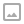## 「BOI2007」Mokia-CDQ分治套CDQ分治

·  ✏️ About  1503 words  ·  ☕ 3 mins read · 👀... views

• 0 W 初始化一个全零矩阵。本命令仅开始时出现一次。
• 1 x y A 向方格 $(x,y)$ 中添加A个用户。A是正整数。
• 2 X1 Y1 X2 Y2 查询 $X1 \leq x \leq X_2$ ， $Y_1 \leq y \leq Y_2$ 所规定的矩形中的用户数量
• 3 无参数 结束程序。本命令仅结束时出现一次。

Luogu P4390

## 题解

1. 对第一维 $a$ 进行排序

1. 对第一维 $a$ 进行分治，递归处理 CDQ1d(L,mid)CDQ1d(mid,R)
2. 按第二维 $b$ 进行归并，此时不计算答案，只记录在这次分治中，该询问/修改属于左半区间 $\text{LEFT}$ 或者 右半区间 $\text{RIGHT}$ 。
3. 复制一份归并后的该区间 $[l,r]$ 的询问数组，用其进行第二维的分治

1. 第二维 $b$ 进行分治，递归处理 CDQ2d(L,mid)CDQ2d(mid,R)
2. 第三维 $c$ 进行归并，此时需要计算答案，记录一个临时变量 $\text{tmp}$ （树状数组）。如果归并左侧新加入的查询/修改在之前维度的分治中均属于左半区间 $\text{LEFT}$ ，则给 $\text{tmp}$ （树状数组）做对应的修改； 如果归并右侧新加入的查询/修改在之前维度的分治中均属于右半区间 $\text{RIGHT}$ ，则计算相关贡献。

## 代码

  1 2 3 4 5 6 7 8 9 10 11 12 13 14 15 16 17 18 19 20 21 22 23 24 25 26 27 28 29 30 31 32 33 34 35 36 37 38 39 40 41 42 43 44 45 46 47 48 49 50 51 52 53 54 55 56 57 58 59 60 61 62 63 64 65 66 67 68 69 70 71 72 73 74 75 76 77 78 79 80 81 82 83 84 85 86 87 88 89 90 91 92 93 94 95 96 97 98 99 100 101 102 103 104  #include using namespace std; const int MAXN = 300000; int n,qaq; struct T{ int id,op,a,b,add,ans,part; //add = 1/-1 T(){ id = op = a = b = add = ans = part = 0; } T(int _id,int _op,int _a,int _b,int _add,int _ans = 0,int _p = 0){ id = _id,op = _op,a = _a,b = _b,add = _add,ans = _ans,part = _p; } }t[MAXN];int tot; int ans[MAXN],vis[MAXN]; int cdq[MAXN],tmp1d[MAXN],tmp2d[MAXN]; const int LEFT = 0,RIGHT = 1; void CDQ2d(int *w,int l,int r){//对第二维(a) 分治，对第三维 (b) 合并 // 这里给出序列 w 的时候应该其第一维坐标 (a) 已经有序 if(l == r) return; int mid = (l+r)>>1; CDQ2d(w,l,mid),CDQ2d(w,mid+1,r);//递归解决子问题 int L = l,R = mid+1,c = l;//现在左边第二维全部小于右边 int tmp = 0; // 跨越维度的分治只需要考虑 (L,b1) 对 (R,b2) 的影响，剩余的在1d的分治里面已经解决 // 更高维度的分治同理，只需要考虑 (L,...,L,b1) 对 (R,...,R,b2) 的影响； // 第一维相同的在 1d 里面解决，第二维相同的在 2d 里面解决 while(c <= r){// 对第三维度进行归并排序 if(R > r || (L<=mid && t[w[L]].b <= t[w[R]].b)){ if(t[w[L]].part == LEFT && t[w[L]].op == 1) tmp += t[w[L]].add; tmp2d[c++] = w[L++]; } else{ if(t[w[R]].part == RIGHT && t[w[R]].op == 2) t[w[R]].ans += tmp; tmp2d[c++] = w[R++]; } } for(int i = l;i<=r;i++) w[i] = tmp2d[i]; } void CDQ1d(int *w,int l,int r){//对第一维（隐去）分治，对第二维合并 if(l == r) return; int mid = (l+r)>>1; CDQ1d(w,l,mid),CDQ1d(w,mid+1,r);// 递归解决子问题 int L = l,R = mid+1,c = l; while(c <= r){ if(R > r || (L <= mid && t[w[L]].a <= t[w[R]].a))// 对第二维进行归并 t[w[L]].part = LEFT,tmp1d[c++] = w[L++]; else t[w[R]].part = RIGHT,tmp1d[c++] = w[R++]; } for(int i = l;i<=r;i++) w[i] = tmp1d[i];// tmp1d相当于复制的一份 CDQ2d(tmp1d,l,r); } void init(){ scanf("%d %d",&qaq,&n); for(int i = 1;;i++){ int op,x,y,x1,y1,v; scanf("%d",&op); if(op == 3){break;} if(op == 1){ scanf("%d %d %d",&x,&y,&v); t[++tot] = T(i,1,x,y,v); } else if(op == 2){ vis[i] = 1; scanf("%d %d %d %d",&x,&y,&x1,&y1); t[++tot] = T(i,2,x-1,y-1,1); t[++tot] = T(i,2,x-1,y1,-1); t[++tot] = T(i,2,x1,y-1,-1); t[++tot] = T(i,2,x1,y1,1); } else{return;} } } void solve(){ for(int i = 1;i<=tot;i++){ cdq[i] = i; } CDQ1d(cdq,1,tot); for(int i = 1;i<=tot;i++){ if(vis[t[i].id]) ans[t[i].id] += t[i].add * t[i].ans; } for(int i = 1;i<=tot;i++){ if(vis[i]){ printf("%d\n",ans[i]); } } } int main(){ init(); solve(); return 0; }WRITTEN BY
cqqqwq
A student in Computer Science.